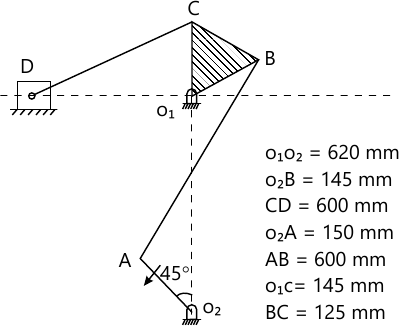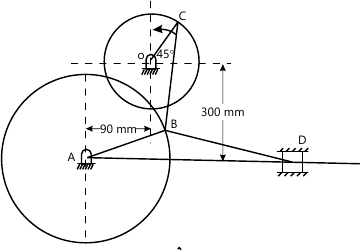MORE IN Theory of Machines - 1
MU Mechanical Engineering (Semester 4)
Theory of Machines - 1
December 2012
Total marks: --
Total time: --
INSTRUCTIONS
(1) Assume appropriate data and state your reasons
(2) Marks are given to the right of every question
(3) Draw neat diagrams wherever necessary

Answer any four of the following:-
1 (a) State Grublers criterion and using the same, deduce minimum number of links required to form a mechanism.
5 M
1 (b) Explain the terms slip and creep as referred to belt drive. On what factors it depends?
5 M
1 (c) D Alembert's principle to bodies under rotational motion about a fixed axis.
5 M
1 (d) What is Coriolis component of acceleration? Explain how the direction is fixed.
5 M
1 (e) Explain law of gearing with a neat sketch.
5 M

2 (a) Derive the condition for correct steering in case of automobile and explain the Ackermans steering gear mechanism with its advantages and correct steering positions.
6 M
2 (b) Figure shows a mechanism having six links. Input link O2A makes an angle 45° with vertical, rotates at an angular velocity w2 of 10 rad/s. Output link D translates in horizontal plane as shown. Determine velocity of slider D using-
i) Relative velocity method
ii) Instantaneous centre method14 M

3 (a)

In the toggle mechanism, as shown in figure is constrained to move on a horizontal path. The dimensions of various links are AB=200 mm, OC=150 mm, BD=450 mm and BC=300 mm. The crank OC is rotating in a counter clockwise direction at a speed of 180 rpm, increasing at the rate of 50 rad/sec2. Find for the given configuration -
i) Velocity and acceleration of D.
ii) Angular velocity and angular acceleration of BD.14 M
3 (b) Sketch a pantograph, explain its working and show that it can be used to reproduce an enlarged scale of a given figure.
6 M

4 (a) A vertical double acting steam engine has cylinder 300 mm diameter and 450 mm stroke and runs at 200 rpm. The reciprocating parts has a mass of 225 kg and the piston rod is 50 mm diameter. The connecting rod is 1.2 m long. When the crank has turned through 125° from the top dead centre, the steam pressure above the piston is 30 kN/m2 and below the piston is 1.5 kN/m2. Calculate the effective turning moment on the crank shaft.
10 M
4 (b) The connecting rod of a gasoline engine is 300 mm long between its centres. It has a mass of 15 kg and mass moment of inertia of 7000 kg-mm2. Its centre of gravity is at 200 mm from its small end centre. Determine the dynamical equivalent two mass system of the connecting rod if one of the masses is located at the small end centre.
10 M

5 (a) A rope drive transmits 600 kW from a pulley of effective diameter 4m, which runs at a speed of 90 r.p.m. The angle of lap is 160°, the angle of groove 45°, the coefficient of friction 0.28, the mass of rope 1.5 kg/m and the allowable tension in each rope 2400 N. Find the number of ropes required.
10 M
5 (b) Two parallel shafts whose centre lines are 4-8 m apart, are connected by an open belt drive. The diameter of the larger pulley is 1.5 m and that of smaller pulley 1m. The initial tension in the belt when stationary is 3 kN. The mass of the belt is 1.5 kg/m length. The coefficient of friction between the belt and pulley is 0.3. Taking centrifugal tension into account, calculate the power transmitted, when the smaller pulley rotates at 400 r.p.m.
10 M

6 (a) Two gear wheels mesh externally and are give velocity ratio of 3 to 1. The teeth are of involute form. Module=6mm, Addendum=one module, Pressure angle=207deg;. The pinion rotates at 90 r.p.m. Determine -
i) The number of teeth on the pinion to avoid interference on it and the corresponding number of teeth on the wheel.
ii) The length of path and arc of contact.
iii) The number of pairs of teeth in contact.
12 M
6 (b) Explain interference in involute gears and to avoid it, find the minimum number of teeth on pinion.
8 M

7 (a) A riveting machine is driven by a constant torque 3 kW motor. The moving parts including the flywheel are equivalent to 150 kg at 0.6 m radius. One riveting operation takes 1 second and absorbs 10,000 N-m of energy. The speed of the flywheel is 300 rpm before riveting. Find the speed immediately after riveting. How many rivets can be closed per minute?
10 M
7 (b) A single cylinder, single acting, four stroke gas engine develops 20 kW at 300 r.p.m. The work done by the gases during the expansion stroke is three times the work done on the gases during the compression stroke, the work done during the suction and exhaust strokes being negligible. If the total fluctuation of speed is not to exceed ±2 percent of the mean and the turning moment diagram during compression and expansion is assumed to be triangular in shape, find the moment of inertia of the flywheel.
10 M

More question papers from Theory of Machines - 1